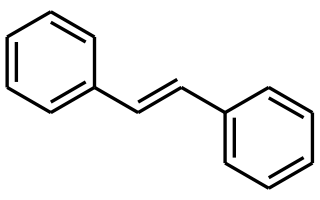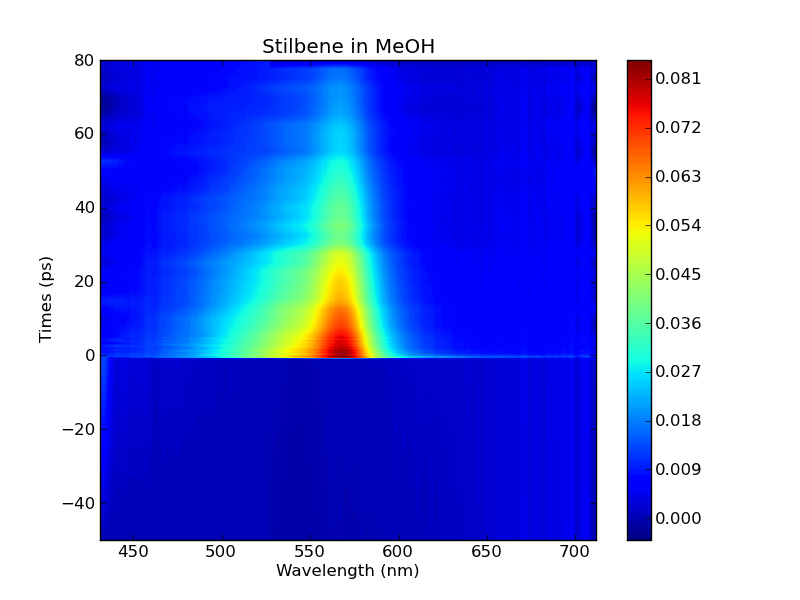## Friday, 29 June 2012

### PyTrA - femtosecond transient absorption data analysis

I have recently written a small program called PyTrA for analyzing data at the Photon Factory at the University of Auckland. The main goals of the program were to to read in data from the Photon Factory software and analyze the time-resolved signals. What it can do so far;
• Import data from the Photon Factory and Ohio State University Center for Chemical and Biophysical Dynamics (CCBD).
• Fix for dispersion (chirp) via polynomial fitting and linear interpolation.
• Fit single traces using pymodelfit.
• Singular value decomposition and reform the spectra of those values.
• Global fitting interface with Igor Pro using win32com (In mac or unix traces saved to folder).
• Visualisation using MayaVI and matplotlib.
• Can export as an csv or time explicit format for analysis in Glotaran or TIMP.
• Basic help window.
I used many different libraries to get the program working thanks to the people that put them together. The GUI is built using TraitsUI provided by Enthought. The fitting is done using pymodelfit which is a model-fitting framework and GUI developed by Erik J Tollerud in TraitsUI using Chaco. Matplotlib and MayaVi provides beautifully rendered figures.

 PyTrA python based TrA data analysis software
I have released the python scripts online at https://sourceforge.net/projects/pytra as an open source distribution. The program is written for python 2.7
The dependencies for PyTrA are;
• Traits
• TraitsUI
• Scipy
• Numpy
• Matplotlib
• MayaVi
These need to be installed separately;
• Pymodelfit
• Setuptools only for windows this sets up the connection with Igor Pro in windows. In other operating systems it just prints out a file into the directory the file was imported from.
Please have a look and tell me if you find any bugs. This is my first bit of coding in python so I would appreciate any advice or helpful additions to the software.

The most exciting part of the project has been the spectral decomposition using singular value decomposition and then reforming the matrices into 2D images which are more easily interpreted.

The data we deal with in transient absorption spectroscopy is of the form

$\Delta A ( \lambda, t) = \sum_{i} A_{i}( \lambda ) e^{-t/\tau_{i}}$

Where $\Delta A$ is our 2D spectrum. Which is made up of different species that have certain corresponding absorptions $A_{i}( \lambda )$ with exponentially decaying time components with $\tau_{i}$ time constant.

A singular value decomposition effectively can be used to analyse the different components of a spectrum. As the different species are linear combinations of their spectra. The form of the decomposition is;
$M = U \Sigma V^{*}$

$A = C \cdot S^{T}$
$U$ is the left singular vectors of M which are eigenvectors of $MM^{*}$ Which is our case corresponds to kinetics.
$\Sigma$ is a diagonal matrix of the singular values which are the square roots of the eigen values of $MM^{*}$ and $M^{*}M$.
$V^{*}$ right singular vectors of M eigenvectors of $M^{*}M$ This is our spectral components.

The common way of viewing this data is by a semi log plot of the singular values which gives you an idea of the contributions of these singular values, a spectral and temporal plot of the right and left singular vectors.

In PyTrA I am playing around with reconstructing 2D plots of the first singular values. This is very useful in analysing the data due to the familarity of these 2D plots with the chemists in the lab. As an example trans-stilbene a photoactive molecule that undergoes a rotationg about the double bond.Stilbene

The spectrum for trans-stilbene in Methanol contains a peak at  ~565 nm which decays with a time constant of  ~50 ps.The SVD plot has going from top to bottom, the number of the vector with a plot of the kinetics below that the value of the singular value 8.52 in this case, a spectral trace and then a reconstructed 2D plot of the component.
Let me know how you find it and if you are able to contribute this is what I have got so far and is just a first release for those who want to have a try out. I want to eventually move over to PyQT to make the interface more interactive and add in multivariate curve resolution.